Ex 5.5

Chapter 5 Class 12 Continuity and Differentiability
Serial order wise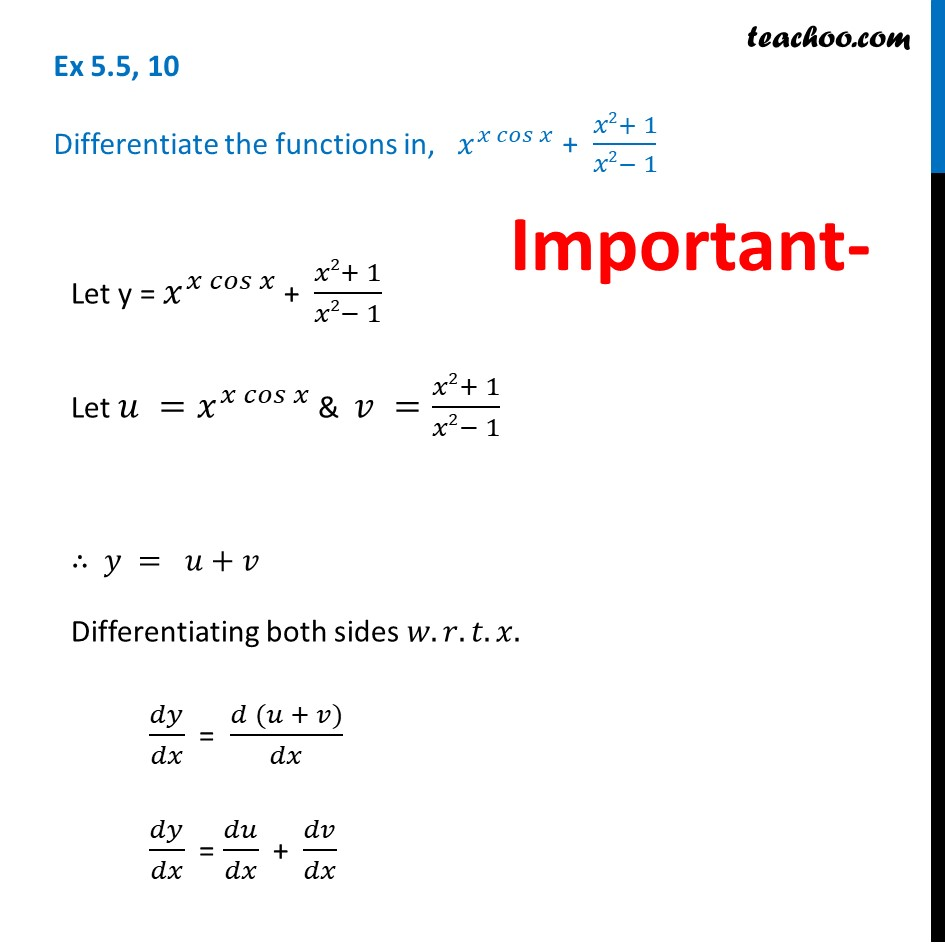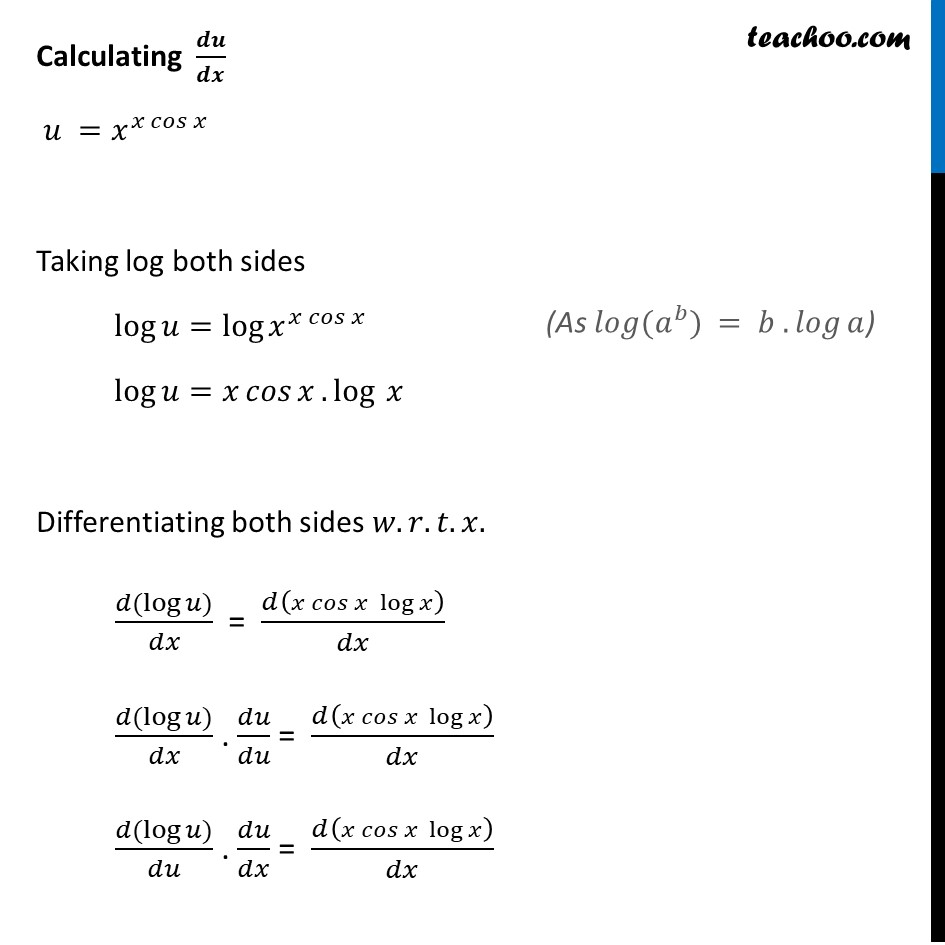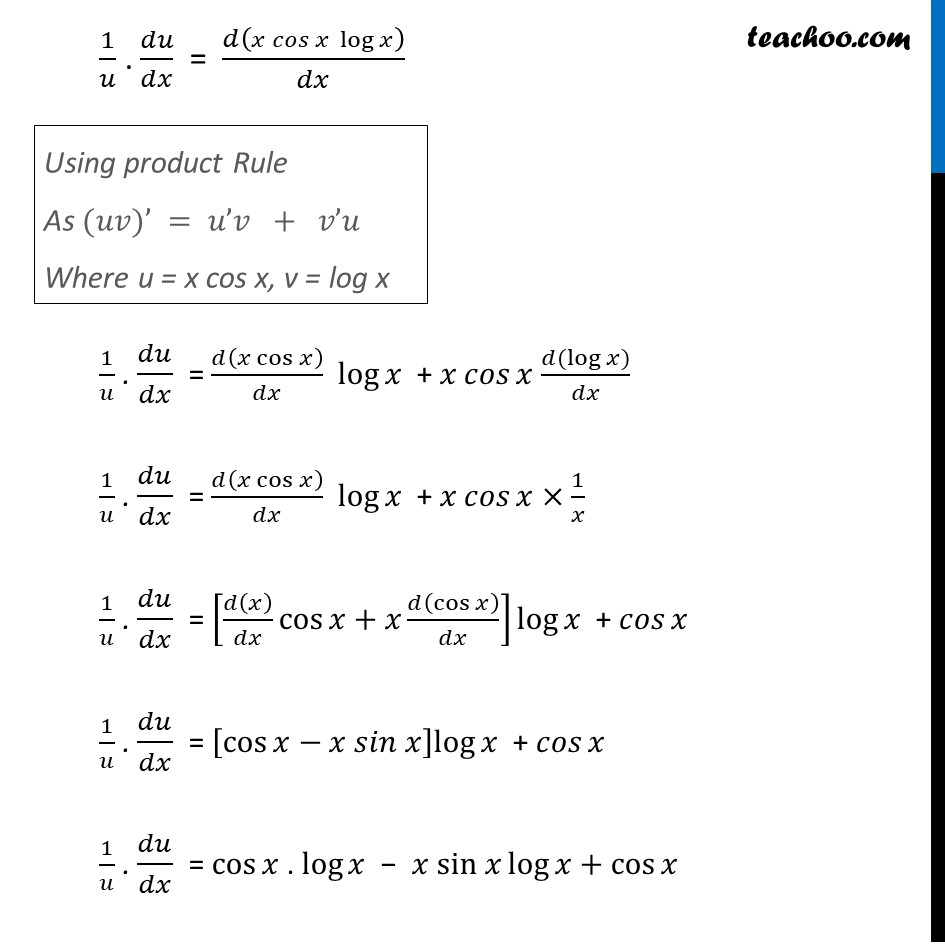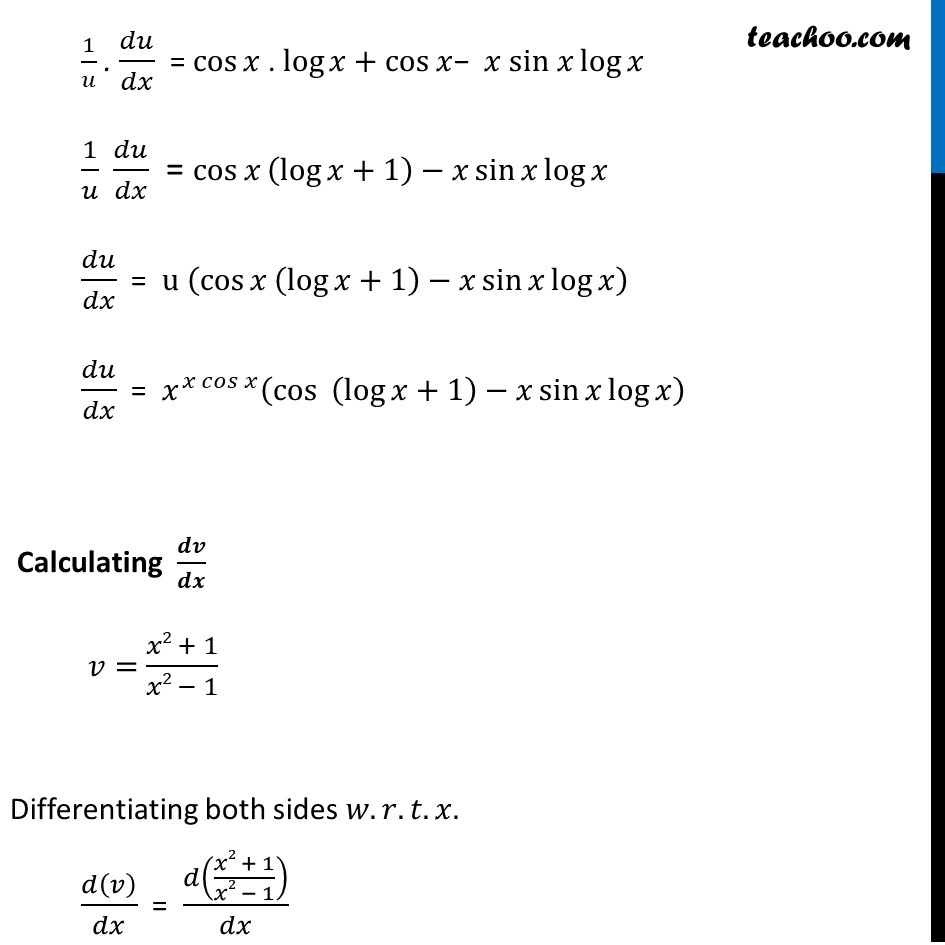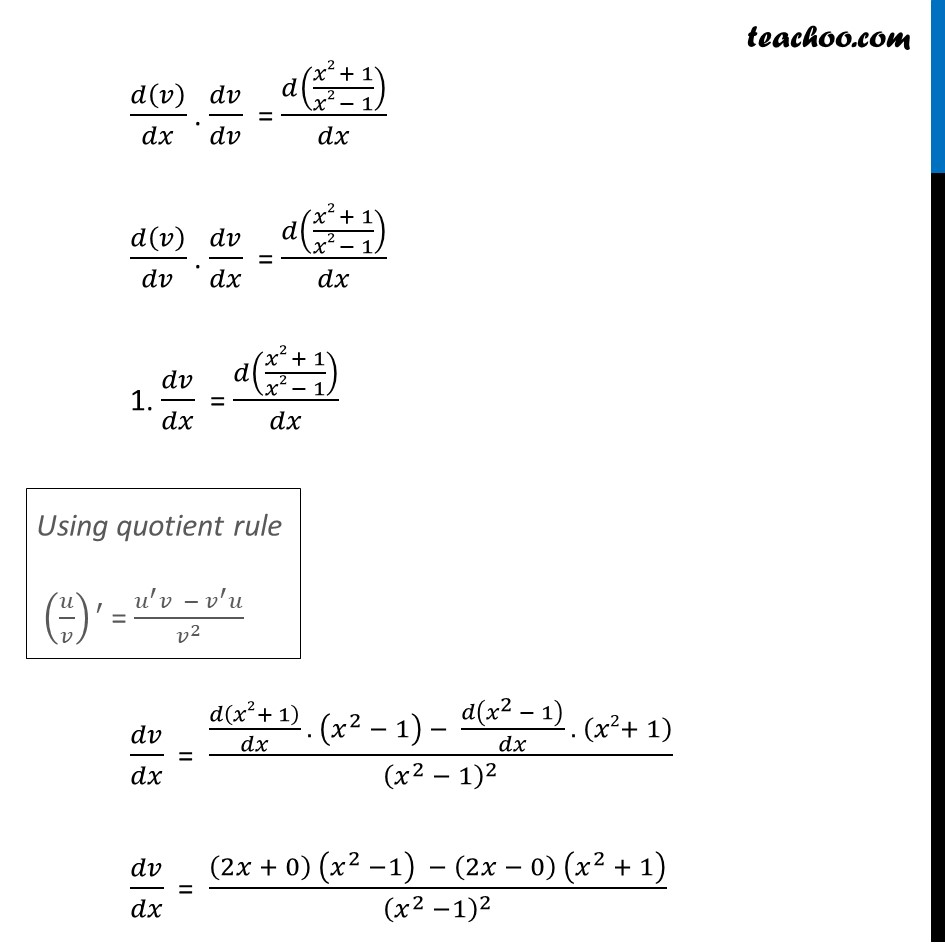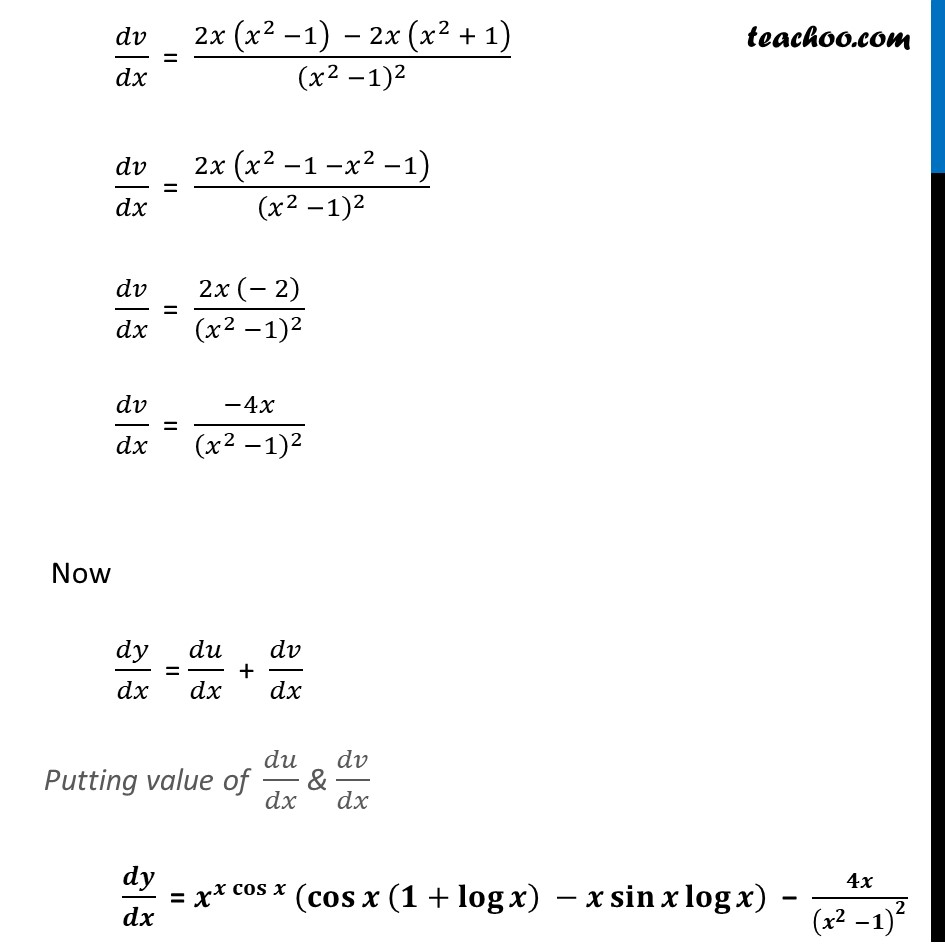Learn in your speed, with individual attention - Teachoo Maths 1-on-1 Class

### Transcript

Ex 5.5, 10 Differentiate the functions in, 𝑥^(𝑥 𝑐𝑜𝑠⁡𝑥 ) + (𝑥2+ 1)/(𝑥2− 1)Let y = 𝑥^(𝑥 𝑐𝑜𝑠⁡𝑥 ) + (𝑥2+ 1)/(𝑥2− 1) Let 𝑢 =𝑥^(𝑥 𝑐𝑜𝑠⁡𝑥 ) & 𝑣 =(𝑥2+ 1)/(𝑥2− 1) ∴ 𝑦 = 𝑢+𝑣 Differentiating both sides 𝑤.𝑟.𝑡.𝑥. 𝑑𝑦/𝑑𝑥 = (𝑑 (𝑢 + 𝑣))/𝑑𝑥 𝑑𝑦/𝑑𝑥 = 𝑑𝑢/𝑑𝑥 + 𝑑𝑣/𝑑𝑥 Calculating 𝒅𝒖/𝒅𝒙 𝑢 =𝑥^(𝑥 𝑐𝑜𝑠⁡𝑥 ) Taking log both sides log⁡𝑢=log⁡〖𝑥^(𝑥 𝑐𝑜𝑠⁡𝑥 ) 〗 log⁡𝑢=𝑥 𝑐𝑜𝑠⁡𝑥. log⁡〖 𝑥〗 Differentiating both sides 𝑤.𝑟.𝑡.𝑥. (𝑑(log⁡𝑢))/𝑑𝑥 = 𝑑(𝑥 𝑐𝑜𝑠⁡𝑥 log⁡𝑥 )/𝑑𝑥 (𝑑(log⁡𝑢))/𝑑𝑥 . 𝑑𝑢/𝑑𝑢 = 𝑑(𝑥 𝑐𝑜𝑠⁡𝑥 log⁡𝑥 )/𝑑𝑥 (𝑑(log⁡𝑢))/𝑑𝑢 . 𝑑𝑢/𝑑𝑥 = 𝑑(𝑥 𝑐𝑜𝑠⁡𝑥 log⁡𝑥 )/𝑑𝑥 (As 𝑙𝑜𝑔⁡(𝑎^𝑏) = 𝑏 . 𝑙𝑜𝑔⁡𝑎) 1/𝑢 . 𝑑𝑢/𝑑𝑥 = 𝑑(𝑥 𝑐𝑜𝑠⁡𝑥 log⁡𝑥 )/𝑑𝑥 1/𝑢 . 𝑑𝑢/𝑑𝑥 = 𝑑(𝑥 cos⁡𝑥 )/𝑑𝑥 log⁡𝑥 + 𝑥 𝑐𝑜𝑠⁡𝑥 (𝑑(log⁡𝑥))/𝑑𝑥 1/𝑢 . 𝑑𝑢/𝑑𝑥 = 𝑑(𝑥 cos⁡𝑥 )/𝑑𝑥 log⁡𝑥 + 𝑥 𝑐𝑜𝑠⁡𝑥 ×1/𝑥 1/𝑢 . 𝑑𝑢/𝑑𝑥 = [𝑑(𝑥)/𝑑𝑥 cos⁡𝑥+𝑥 𝑑(cos⁡𝑥 )/𝑑𝑥]log⁡𝑥 + 𝑐𝑜𝑠⁡𝑥 1/𝑢 . 𝑑𝑢/𝑑𝑥 = [cos⁡𝑥−𝑥 𝑠𝑖𝑛 𝑥]log⁡𝑥 + 𝑐𝑜𝑠⁡𝑥 1/𝑢 . 𝑑𝑢/𝑑𝑥 = cos⁡𝑥 . log⁡𝑥 − 𝑥 sin 𝑥 log⁡𝑥+cos⁡𝑥 Using product Rule As (𝑢𝑣)’ = 𝑢’𝑣 + 𝑣’𝑢 Where u = x cos x, v = log x 1/𝑢 . 𝑑𝑢/𝑑𝑥 = cos⁡𝑥 . log⁡𝑥+cos⁡𝑥− 𝑥 sin 𝑥 log⁡𝑥 1/𝑢 𝑑𝑢/𝑑𝑥 = cos⁡𝑥 (log⁡𝑥+1)−𝑥 sin⁡〖𝑥 log⁡𝑥 〗 𝑑𝑢/𝑑𝑥 = u (cos⁡𝑥 (log⁡𝑥+1)−𝑥 sin⁡〖𝑥 log⁡𝑥 〗 ) 𝑑𝑢/𝑑𝑥 = 𝑥^(𝑥 𝑐𝑜𝑠⁡𝑥 ) (cos⁡〖 (log⁡〖𝑥+1〗 )−𝑥 sin⁡〖𝑥 log⁡𝑥 〗 〗 ) Calculating 𝒅𝒗/𝒅𝒙 𝑣= (𝑥2 + 1)/(𝑥2 − 1) Differentiating both sides 𝑤.𝑟.𝑡.𝑥. 𝑑(𝑣)/𝑑𝑥 = 𝑑((𝑥2 + 1)/(𝑥2 − 1))/𝑑𝑥 𝑑(𝑣)/𝑑𝑥 . 𝑑𝑣/𝑑𝑣 = 𝑑((𝑥2 + 1)/(𝑥2 − 1))/𝑑𝑥 𝑑(𝑣)/𝑑𝑣 . 𝑑𝑣/𝑑𝑥 = 𝑑((𝑥2 + 1)/(𝑥2 − 1))/𝑑𝑥 1. 𝑑𝑣/𝑑𝑥 = 𝑑((𝑥2 + 1)/(𝑥2 − 1))/𝑑𝑥 𝑑𝑣/𝑑𝑥 = (𝑑(𝑥2+ 1)/𝑑𝑥 . (𝑥^2 − 1) − 𝑑(𝑥^2 − 1)/𝑑𝑥 . (𝑥2+ 1))/(𝑥^2 − 1)^2 𝑑𝑣/𝑑𝑥 = ((2𝑥 + 0) (𝑥^2 −1) − (2𝑥 − 0) (𝑥^2 + 1))/(𝑥^2 −1)^2 Using quotient rule (𝑢/𝑣)′ = (𝑢^′ 𝑣 − 𝑣^′ 𝑢)/𝑣^2 𝑑𝑣/𝑑𝑥 = (2𝑥 (𝑥^2 −1) − 2𝑥 (𝑥^2 + 1))/(𝑥^2 −1)^2 𝑑𝑣/𝑑𝑥 = (2𝑥 (𝑥^2 −1 −𝑥^2 −1))/(𝑥^2 −1)^2 𝑑𝑣/𝑑𝑥 = (2𝑥 (− 2))/(𝑥^2 −1)^2 𝑑𝑣/𝑑𝑥 = (−4𝑥)/(𝑥^2 −1)^2 Now 𝑑𝑦/𝑑𝑥 = 𝑑𝑢/𝑑𝑥 + 𝑑𝑣/𝑑𝑥 Putting value of 𝑑𝑢/𝑑𝑥 & 𝑑𝑣/𝑑𝑥 𝒅𝒚/𝒅𝒙 = 𝒙^〖𝒙 𝐜𝐨𝐬〗⁡𝒙 (𝐜𝐨𝐬⁡〖𝒙 (𝟏+𝐥𝐨𝐠⁡𝒙 ) −𝒙 𝐬𝐢𝐧⁡〖𝒙 𝐥𝐨𝐠⁡𝒙 〗 〗 ) − 𝟒𝒙/(𝒙^𝟐 −𝟏)^𝟐